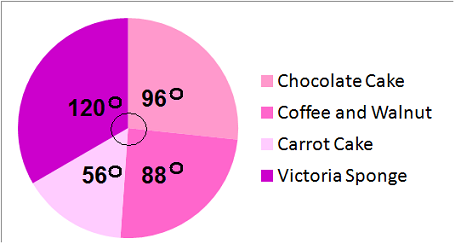### Pie Charts

Pie charts are circles split into sections

To draw a pie chart:

 (a) Find the total frequency (b) Find the angle of one frequency: 360 degrees/total frequency (c) Find the degrees of each section: angle of one frequency × no of items in one section (d) Draw the pie chart

#### Example 1.   Draw a Pie Chart of the number of cakes sold

 Cake Frequency f Chocolate Cake 24 Coffee & Walnut 22 Carrot Cake 14 Victoria Sponge 30

(a)   Add up the frequencies to work out the total

 Cake Frequency f Chocolate Cake 24 Coffee & Walnut 22 Carrot Cake 14 Victoria Sponge 30 Total 90

(b)   Find the angle of one cake:

Angle of one cake sold: 360 degrees / total frequency
Angle of one cake: 360/90 = 4

(c)   Find the degrees of each section:

 Cake f angle foreach section Chocolate Cake 24 24 × 4 = 96 Coffee & Walnut 22 22 × 4 = 88 Carrot Cake 14 14 × 4 = 56 Victoria Sponge 30 30 × 4 = 120 Total 90 90 × 4 = 360

(d)   Draw the Pie Chart:to: